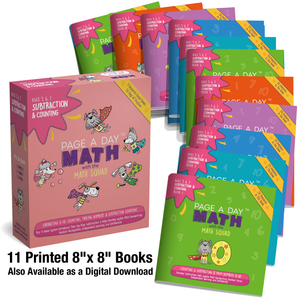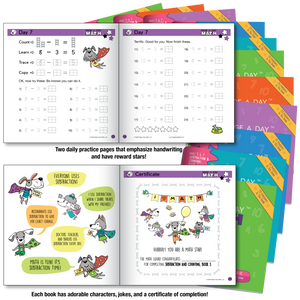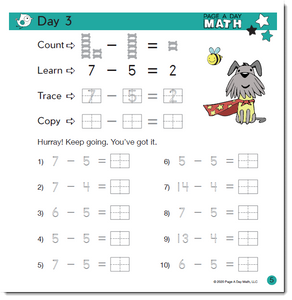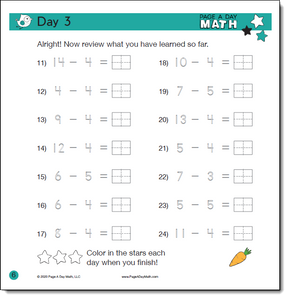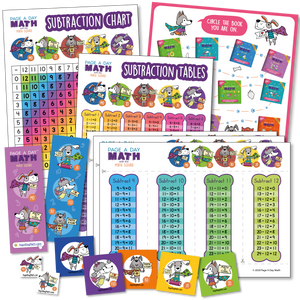# SUBTRACTION | 11 Subtraction Workbooks

\$79.95
\$79.95

The First Individualized Math Learning System, Like Kumon, That Allows Every Student To Study at the "Just Right" Level...from home.

What You'll Get:

1. Subtraction Program - 11 Books, 6 months of practice.
2. Progress Tracker
3. Subtraction Chart
4. Subtraction Table
5. Subtraction Activity
6. Stickers, Bookmarks, Tattoos

Perfect for young students starting subtraction, often in K-2nd grade, developing basic subtraction fact fluency and math handwriting
Learning objectives Subtract numbers 0-10 up to 20-10=10, learn one new subtraction fact daily, recall facts with fluency, develop legible handwriting, number sense, and confidence.
Each book has 28 practice pages, one new subtraction fact daily (dog bones for counting to make subtraction fun), 24 daily problems, 14 days of practice, 350 problems per book, a completion certificate at the end of every book, daily achievements stars, dog joke on every back cover, handwriting aids, and cumulative practice.
This subtraction math kit has 10 books with step-by-step subtraction development, practice, and repetition to last 140 days, fun & easy to use curriculum, small size for young hands, repetition for fluency. Over 3,500 practice problems in all.

*This Subtraction Kit comes without an orange outer box.